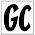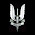### Analyzing Randomly Descending Sequences

Finals week just ended for me so I'm hoping to post some new cool math problems in the next few days.  In this blog post, I present a math problem that is very easy to understand what it is asking, but not so easy to solve.  Regardless, I will present my solution, which yielded a very interesting result.
You construct a sequence $k_1, k_2, ..., k_m$ by randomly picking an integer from the set $0 \leq k_1 < n$.  After picking $k_1$, you repeat the process by picking $k_2$ randomly from $0 \leq k_2 < k_1$ and repeat the process until you can no longer pick any numbers (when $k_m = 0$).  Find a function for $f(n)$, where $f(n)$ is the expected value of the length of this sequence.

### Comments

1.2.3.Andaman and Nicobar Islands govt jobs

4.5.This comment has been removed by the author.

6.This comment has been removed by the author.

7.thanks for the proper explanation, I was quite confused how to post a reply. Koora